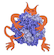IMP  2.4.0 The Integrative Modeling Platform
Go to the documentation of this file.
1 /**
4  *
5  * WARNING This file was generated from QuadScore.h
6  * in /tmp/nightly-build-65532/imp-2.4.0/tools/build/container_templates/kernel
7  * by tools/build/make_containers.py.
8  *
10  */
11
14
15 #include <IMP/kernel/kernel_config.h>
16 #include "base_types.h"
17 #include "ParticleTuple.h"
18 #include "DerivativeAccumulator.h"
19 #include "internal/container_helpers.h"
21 #include "model_object_helpers.h"
22
23 IMPKERNEL_BEGIN_NAMESPACE
24
25 //! Abstract class for scoring object(s) of type ParticleQuad
26 /** QuadScore will evaluate the score and derivatives
27  for passed object(s) of type ParticleQuad.
28
29  Use in conjunction with various
30  restraints such as IMP::core::QuadsRestraint or
31  IMP::core::QuadRestraint. The restraints couple the score
32  functions with appropariate lists of object(s) of type ParticleQuad.
33
34  Implementers should check out IMP_QUAD_SCORE().
35
37 */
38 class IMPKERNELEXPORT QuadScore : public ParticleInputs,
39  public base::Object {
40  public:
47  //! Compute the score and the derivative if needed.
48  /** \deprecated_at{2.1} Use the index-based evaluate instead. */
49  IMPKERNEL_DEPRECATED_METHOD_DECL(2.1)
50  virtual double evaluate(const kernel::ParticleQuad& vt, DerivativeAccumulator *da) const;
51
52  //! Compute the score and the derivative if needed.
53  /** evaluate the score and the derivative if needed over vt
54
55  @param m the model of vt
56  @param vt the index in m of an object of type ParticleQuad
57  @param da a derivative accumulator that reweighting
58  computed derivatives. If nullptr, derivatives
59  will not be computed
60  */
61  virtual double evaluate_index(kernel::Model *m, const kernel::ParticleIndexQuad& vt,
62  DerivativeAccumulator *da) const;
63
64  //! Compute the score and the derivative if needed over a set.
65  /** evaluate the score and the derivative if needed over a set
66  of objects in o
67
68  @param m the model of o
69  @param o objects of type ParticleQuad, specified by index
70  @param da a derivative accumulator that reweighting
71  computed derivatives. If nullptr, derivatives
72  will not be computed
73  @param lower_bound index of first item in o to evaluate
74  @param upper_bound index of last item in o to evaluate
75
76  @note Implementations
77  for these are provided by the IMP_QUAD_SCORE()
78  macro.
79  */
80  virtual double evaluate_indexes(kernel::Model *m, const kernel::ParticleIndexQuads &o,
82  unsigned int lower_bound,
83  unsigned int upper_bound) const;
84
85  //! Compute the score and the derivative if needed.
86  /** Compute the score and the derivative if needed as in evaluate_index().
87  but may terminate the computation early if the score is higher than max.
88
89  @return the score if score<= max or some arbitrary value > max otherwise.
90  */
91  virtual double evaluate_if_good_index(kernel::Model *m, const kernel::ParticleIndexQuad& vt,
93  double max) const;
94
95  /** Compute the score and the derivative if needed as in evaluate_index().
96  but may terminate the computation early if the total score is higher than max.
97
98  @return the score if score<= max or some arbitrary value > max otherwise.
99
100  Implementations
101  for these are provided by the IMP_QUAD_SCORE()
102  macro.
103  */
104  virtual double evaluate_if_good_indexes(kernel::Model *m,
106  DerivativeAccumulator *da, double max,
107  unsigned int lower_bound,
108  unsigned int upper_bound) const;
109  /** Decompose this pair score acting on the pair into a set of
110  restraints. The scoring function and derivatives should
111  be equal to the current score. The defualt implementation
112  just returns this object bound to the pair.*/
113  Restraints create_current_decomposition(kernel::Model *m,
115
116  protected:
117  /** Overide this to return your own decomposition.*/
118  virtual Restraints do_create_current_decomposition(kernel::Model *m,
120
122 };
123
124 IMPKERNEL_END_NAMESPACE
125
Class for adding derivatives from restraints to the model.
Abstract class for scoring object(s) of type ParticleQuad.
Class for adding derivatives from restraints to the model.
Basic types used by IMP.